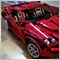Need help for indicator11

Hello, I'm trying to develop an indicator that analyzes on the M30 chart when two MAs cross and then shows it on the H1 chart, but it doesn't work for me.

```#property indicator_chart_window
#property indicator_buffers 4
#property indicator_plots 2

#property indicator_label1 "Up_Period30"
#property indicator_type1 DRAW_ARROW
#property indicator_color1 clrBlue

#property indicator_label2 "Down_Period30"
#property indicator_type2 DRAW_ARROW
#property indicator_color2 clrRed

int MovingAverage12_Period30;
int MovingAverage22_Period30;

double MovingAverageBuffer12_Period30[];
double MovingAverageBuffer22_Period30[];

double UpBuffer_Period30[];
double DownBuffer_Period30[];
//+------------------------------------------------------------------+
//| Custom indicator initialization function                         |
//+------------------------------------------------------------------+
int OnInit()
{
SetIndexBuffer(0,UpBuffer_Period30,INDICATOR_DATA);
SetIndexBuffer(1,DownBuffer_Period30,INDICATOR_DATA);
SetIndexBuffer(2,MovingAverageBuffer12_Period30,INDICATOR_CALCULATIONS);
SetIndexBuffer(3,MovingAverageBuffer22_Period30,INDICATOR_CALCULATIONS);

MovingAverage12_Period30=iMA(_Symbol,PERIOD_M30,12,0,MODE_EMA,PRICE_OPEN);
if(MovingAverage12_Period30==INVALID_HANDLE)
{
Print("The iMA object was not created: Error ",GetLastError());
return INIT_FAILED;
}

MovingAverage22_Period30=iMA(_Symbol,PERIOD_M30,22,0,MODE_EMA,PRICE_OPEN);
if(MovingAverage22_Period30==INVALID_HANDLE)
{
Print("The iMA object was not created: Error ",GetLastError());
return INIT_FAILED;
}

return(INIT_SUCCEEDED);
}
//+------------------------------------------------------------------+
//| Custom indicator iteration function                              |
//+------------------------------------------------------------------+
int OnCalculate(const int rates_total,
const int prev_calculated,
const datetime &time[],
const double &open[],
const double &high[],
const double &low[],
const double &close[],
const long &tick_volume[],
const long &volume[],
{
ArraySetAsSeries(high,true);
ArraySetAsSeries(low,true);
ArraySetAsSeries(UpBuffer_Period30,true);
ArraySetAsSeries(DownBuffer_Period30,true);

int limit=MathMin(rates_total-1,rates_total-prev_calculated);

int copied12_Period30=CopyBuffer(MovingAverage12_Period30,0,0,limit,MovingAverageBuffer12_Period30);
int copied22_Period30=CopyBuffer(MovingAverage22_Period30,0,0,limit,MovingAverageBuffer22_Period30);

for(int i=0;i<limit;i++)
{
UpBuffer_Period30[i]=EMPTY_VALUE;
DownBuffer_Period30[i]=EMPTY_VALUE;

if(_Period==PERIOD_H1)
{
if(MovingAverageBuffer12_Period30[i]>MovingAverageBuffer22_Period30[i] && MovingAverageBuffer12_Period30[i+1]<MovingAverageBuffer22_Period30[i+1])
{
datetime CandleTime=iTime(_Symbol,PERIOD_M30,i);

int Bar=iBarShift(_Symbol,_Period,CandleTime,true);

UpBuffer_Period30[Bar]=low[Bar]-0.00030;
}
else if(MovingAverageBuffer12_Period30[i]<MovingAverageBuffer22_Period30[i] && MovingAverageBuffer12_Period30[i+1]>MovingAverageBuffer22_Period30[i+1])
{
datetime CandleTime=iTime(_Symbol,PERIOD_M30,i);

int Bar=iBarShift(_Symbol,_Period,CandleTime,true);

DownBuffer_Period30[Bar]=high[Bar]+0.00030;
}
}
}

return(rates_total);
}
//+------------------------------------------------------------------+
```563

Baxter9311:

Hello, I'm trying to develop an indicator that analyzes on the M30 chart when two MAs cross and then shows it on the H1 chart, but it doesn't work for me.

There are some issues with this block:

```         if(MovingAverageBuffer12_Period30[i]>MovingAverageBuffer22_Period30[i] && MovingAverageBuffer12_Period30[i+1]<MovingAverageBuffer22_Period30[i+1])
{
datetime CandleTime=iTime(_Symbol,PERIOD_M30,i);

int Bar=iBarShift(_Symbol,_Period,CandleTime,true);

UpBuffer_Period30[Bar]=low[Bar]-0.00030;
}
else if(MovingAverageBuffer12_Period30[i]<MovingAverageBuffer22_Period30[i] && MovingAverageBuffer12_Period30[i+1]>MovingAverageBuffer22_Period30[i+1])
{
datetime CandleTime=iTime(_Symbol,PERIOD_M30,i);

int Bar=iBarShift(_Symbol,_Period,CandleTime,true);

DownBuffer_Period30[Bar]=high[Bar]+0.00030;
}
```

(1) Your i is based on _Period (since it is based on rates_total and prev_calculated), and your MovingAverageBuffers and iTime() for PERIOD_M30 uses i - the same i for different periods will give you different times and your result will be garbage.

(2) You need to check the return values of iBarShift(), because if the time is outside of all the available candles, iBarShift() returns -1, and will cause the next line to fail (array out of range).11

Honestly, I don't get it.563

Baxter9311:
Honestly, I don't get it.

Then look at this table that illustrates my two points above - assuming current time is 1200 hrs:

iTime (T60) at Bar i on H1 Chart
(i.e. iTime(_Symbol,_Period,i)
Time (T30) at Bar i on M30 Chart
(i.e. iTime(_Symbol,PERIOD_M30,i)
Bar
(i.e. iBarShift(_Symbol,_Period,T30,true))
0120012000
111001130-1 (out of range)
2100011001
309001030-1 (out of range)
4080010002
507000930-1 (out of range)
6060009003
::::
10020007005

Notice that T60 and T30 are not the same for the same bar number i.18880

1. Seng Joo Thio:

Then look at this table that illustrates my two points above - assuming current time is 1200 hrs: …

Notice that T60 and T30 are not the same for the same bar number i.

Correct
1. If you pass 1130 to iBarShift(H1) you will get -1 with true. So stop using true, use the default and you'll get the H1 shift for the bar containing 1130. M30 shift one is 1130, H1 shift one is 1100. M30 shift 3 is 1030, H1 shift 2 is 1000.
2. Not, in general.

2. Baxter9311: Honestly, I don't get it.
Don't mix apples and oranges.